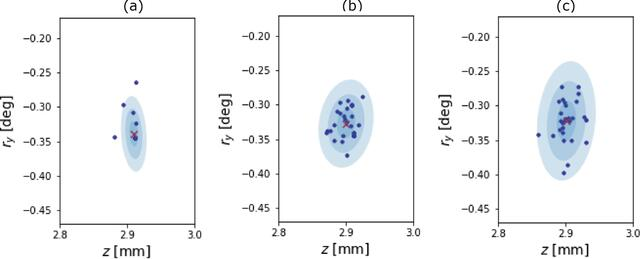disable zoom     view article Figure 7 Depicted are the spatial results of three Monte Carlo simulations, in two of the four total dimensions. We varied the additive noise parameter ς between each sampling. Panel (a) depicts the case where ς = 5.0 × 10−4, (b) shows the case where ς = 5.0 × 10−3, and (c) shows the ς = 5.0 × 10−2 case. All results are depicted as blue scatter points above three blue confidence regions. The mean result is presented as a red cross.JOURNAL OFSYNCHROTRONRADIATION
ISSN: 1600-5775
Volume 29| Part 4| July 2022| Pages 947-956